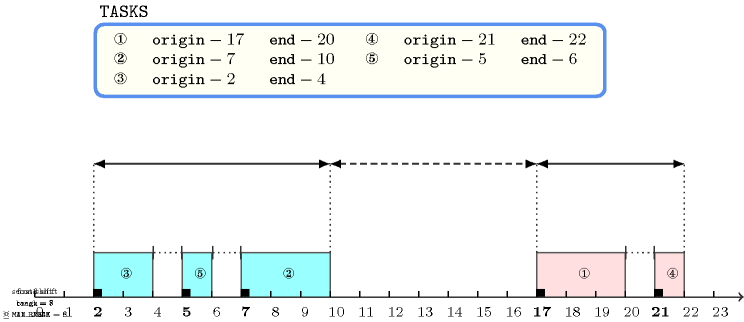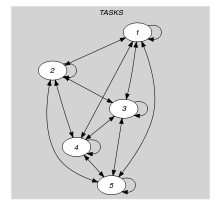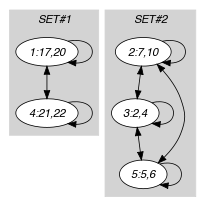## 5.345. shift

Origin

N. Beldiceanu

Constraint

$\mathrm{𝚜𝚑𝚒𝚏𝚝}\left(\mathrm{𝙼𝙸𝙽}_\mathrm{𝙱𝚁𝙴𝙰𝙺},\mathrm{𝙼𝙰𝚇}_\mathrm{𝚁𝙰𝙽𝙶𝙴},\mathrm{𝚃𝙰𝚂𝙺𝚂}\right)$

Arguments
 $\mathrm{𝙼𝙸𝙽}_\mathrm{𝙱𝚁𝙴𝙰𝙺}$ $\mathrm{𝚒𝚗𝚝}$ $\mathrm{𝙼𝙰𝚇}_\mathrm{𝚁𝙰𝙽𝙶𝙴}$ $\mathrm{𝚒𝚗𝚝}$ $\mathrm{𝚃𝙰𝚂𝙺𝚂}$ $\mathrm{𝚌𝚘𝚕𝚕𝚎𝚌𝚝𝚒𝚘𝚗}\left(\mathrm{𝚘𝚛𝚒𝚐𝚒𝚗}-\mathrm{𝚍𝚟𝚊𝚛},\mathrm{𝚎𝚗𝚍}-\mathrm{𝚍𝚟𝚊𝚛}\right)$
Restrictions
 $\mathrm{𝙼𝙸𝙽}_\mathrm{𝙱𝚁𝙴𝙰𝙺}>0$ $\mathrm{𝙼𝙰𝚇}_\mathrm{𝚁𝙰𝙽𝙶𝙴}>0$ $\mathrm{𝚛𝚎𝚚𝚞𝚒𝚛𝚎𝚍}$$\left(\mathrm{𝚃𝙰𝚂𝙺𝚂},\left[\mathrm{𝚘𝚛𝚒𝚐𝚒𝚗},\mathrm{𝚎𝚗𝚍}\right]\right)$ $\mathrm{𝚃𝙰𝚂𝙺𝚂}.\mathrm{𝚘𝚛𝚒𝚐𝚒𝚗}<\mathrm{𝚃𝙰𝚂𝙺𝚂}.\mathrm{𝚎𝚗𝚍}$
Purpose

The difference between the end of the last task of a shift and the origin of the first task of a shift should not exceed the quantity $\mathrm{𝙼𝙰𝚇}_\mathrm{𝚁𝙰𝙽𝙶𝙴}$. Two tasks ${t}_{1}$ and ${t}_{2}$ belong to the same shift if at least one of the following conditions is true:

• Task ${t}_{2}$ starts after the end of task ${t}_{1}$ at a distance that is less than or equal to the quantity $\mathrm{𝙼𝙸𝙽}_\mathrm{𝙱𝚁𝙴𝙰𝙺}$,

• Task ${t}_{1}$ starts after the end of task ${t}_{2}$ at a distance that is less than or equal to the quantity $\mathrm{𝙼𝙸𝙽}_\mathrm{𝙱𝚁𝙴𝙰𝙺}$.

• Task ${t}_{1}$ overlaps task ${t}_{2}$.

Example
$\left(\begin{array}{c}6,8,〈\begin{array}{cc}\mathrm{𝚘𝚛𝚒𝚐𝚒𝚗}-17\hfill & \mathrm{𝚎𝚗𝚍}-20,\hfill \\ \mathrm{𝚘𝚛𝚒𝚐𝚒𝚗}-7\hfill & \mathrm{𝚎𝚗𝚍}-10,\hfill \\ \mathrm{𝚘𝚛𝚒𝚐𝚒𝚗}-2\hfill & \mathrm{𝚎𝚗𝚍}-4,\hfill \\ \mathrm{𝚘𝚛𝚒𝚐𝚒𝚗}-21\hfill & \mathrm{𝚎𝚗𝚍}-22,\hfill \\ \mathrm{𝚘𝚛𝚒𝚐𝚒𝚗}-5\hfill & \mathrm{𝚎𝚗𝚍}-6\hfill \end{array}〉\hfill \end{array}\right)$

Figure 5.345.1 represents the different tasks of the example. Each task is drawn as a rectangle with its corresponding $\mathrm{𝚒𝚍}$ attribute in the middle. We indicate the distance between two consecutive tasks of a same shift and note that it is less than or equal to $\mathrm{𝙼𝙸𝙽}_\mathrm{𝙱𝚁𝙴𝙰𝙺}=6$. Since each shift has a range that is less than or equal to $\mathrm{𝙼𝙰𝚇}_\mathrm{𝚁𝙰𝙽𝙶𝙴}=8$, the $\mathrm{𝚜𝚑𝚒𝚏𝚝}$ constraint holds (the range of a shift is the difference between the end of the last task of the shift and the origin of the first task of the shift).

##### Figure 5.345.1. The two shifts of the Example slotTypical
 $\mathrm{𝙼𝙸𝙽}_\mathrm{𝙱𝚁𝙴𝙰𝙺}>1$ $\mathrm{𝙼𝙰𝚇}_\mathrm{𝚁𝙰𝙽𝙶𝙴}>1$ $\mathrm{𝙼𝙸𝙽}_\mathrm{𝙱𝚁𝙴𝙰𝙺}<\mathrm{𝙼𝙰𝚇}_\mathrm{𝚁𝙰𝙽𝙶𝙴}$ $|\mathrm{𝚃𝙰𝚂𝙺𝚂}|>2$
Symmetries
• Items of $\mathrm{𝚃𝙰𝚂𝙺𝚂}$ are permutable.

• One and the same constant can be added to the $\mathrm{𝚘𝚛𝚒𝚐𝚒𝚗}$ attribute of all items of $\mathrm{𝚃𝙰𝚂𝙺𝚂}$.

Usage

The shift constraint can be used in machine scheduling problems where one has to shut down a machine for maintenance purpose after a given maximum utilisation of that machine. In this case the $\mathrm{𝙼𝙰𝚇}_\mathrm{𝚁𝙰𝙽𝙶𝙴}$ parameter indicates the maximum possible utilisation of the machine before maintenance, while the $\mathrm{𝙼𝙸𝙽}_\mathrm{𝙱𝚁𝙴𝙰𝙺}$ parameter gives the minimum time needed for maintenance.

The shift constraint can also be used for timetabling problems where the rest period of a person can move in time. In this case $\mathrm{𝙼𝙰𝚇}_\mathrm{𝚁𝙰𝙽𝙶𝙴}$ indicates the maximum possible working time for a person, while $\mathrm{𝙼𝙸𝙽}_\mathrm{𝙱𝚁𝙴𝙰𝙺}$ specifies the minimum length of the break that follows a working time period.

Keywords
Arc input(s)

$\mathrm{𝚃𝙰𝚂𝙺𝚂}$

Arc generator
$\mathrm{𝑆𝐸𝐿𝐹}$$↦\mathrm{𝚌𝚘𝚕𝚕𝚎𝚌𝚝𝚒𝚘𝚗}\left(\mathrm{𝚝𝚊𝚜𝚔𝚜}\right)$

Arc arity
Arc constraint(s)
 $•\mathrm{𝚝𝚊𝚜𝚔𝚜}.\mathrm{𝚎𝚗𝚍}\ge \mathrm{𝚝𝚊𝚜𝚔𝚜}.\mathrm{𝚘𝚛𝚒𝚐𝚒𝚗}$ $•\mathrm{𝚝𝚊𝚜𝚔𝚜}.\mathrm{𝚎𝚗𝚍}-\mathrm{𝚝𝚊𝚜𝚔𝚜}.\mathrm{𝚘𝚛𝚒𝚐𝚒𝚗}\le \mathrm{𝙼𝙰𝚇}_\mathrm{𝚁𝙰𝙽𝙶𝙴}$
Graph property(ies)
$\mathrm{𝐍𝐀𝐑𝐂}$$=|\mathrm{𝚃𝙰𝚂𝙺𝚂}|$

Arc input(s)

$\mathrm{𝚃𝙰𝚂𝙺𝚂}$

Arc generator
$\mathrm{𝐶𝐿𝐼𝑄𝑈𝐸}$$↦\mathrm{𝚌𝚘𝚕𝚕𝚎𝚌𝚝𝚒𝚘𝚗}\left(\mathrm{𝚝𝚊𝚜𝚔𝚜}\mathtt{1},\mathrm{𝚝𝚊𝚜𝚔𝚜}\mathtt{2}\right)$

Arc arity
Arc constraint(s)
$\bigvee \left(\begin{array}{c}\bigwedge \left(\begin{array}{c}\mathrm{𝚝𝚊𝚜𝚔𝚜}\mathtt{2}.\mathrm{𝚘𝚛𝚒𝚐𝚒𝚗}\ge \mathrm{𝚝𝚊𝚜𝚔𝚜}\mathtt{1}.\mathrm{𝚎𝚗𝚍},\hfill \\ \mathrm{𝚝𝚊𝚜𝚔𝚜}\mathtt{2}.\mathrm{𝚘𝚛𝚒𝚐𝚒𝚗}-\mathrm{𝚝𝚊𝚜𝚔𝚜}\mathtt{1}.\mathrm{𝚎𝚗𝚍}\le \mathrm{𝙼𝙸𝙽}_\mathrm{𝙱𝚁𝙴𝙰𝙺}\hfill \end{array}\right),\hfill \\ \bigwedge \left(\begin{array}{c}\mathrm{𝚝𝚊𝚜𝚔𝚜}\mathtt{1}.\mathrm{𝚘𝚛𝚒𝚐𝚒𝚗}\ge \mathrm{𝚝𝚊𝚜𝚔𝚜}\mathtt{2}.\mathrm{𝚎𝚗𝚍},\hfill \\ \mathrm{𝚝𝚊𝚜𝚔𝚜}\mathtt{1}.\mathrm{𝚘𝚛𝚒𝚐𝚒𝚗}-\mathrm{𝚝𝚊𝚜𝚔𝚜}\mathtt{2}.\mathrm{𝚎𝚗𝚍}\le \mathrm{𝙼𝙸𝙽}_\mathrm{𝙱𝚁𝙴𝙰𝙺}\hfill \end{array}\right),\hfill \\ \bigwedge \left(\begin{array}{c}\mathrm{𝚝𝚊𝚜𝚔𝚜}\mathtt{2}.\mathrm{𝚘𝚛𝚒𝚐𝚒𝚗}<\mathrm{𝚝𝚊𝚜𝚔𝚜}\mathtt{1}.\mathrm{𝚎𝚗𝚍},\hfill \\ \mathrm{𝚝𝚊𝚜𝚔𝚜}\mathtt{1}.\mathrm{𝚘𝚛𝚒𝚐𝚒𝚗}<\mathrm{𝚝𝚊𝚜𝚔𝚜}\mathtt{2}.\mathrm{𝚎𝚗𝚍}\hfill \end{array}\right)\hfill \end{array}\right)$
Sets
$\begin{array}{c}\mathrm{𝖢𝖢}↦\hfill \\ \left[\begin{array}{c}\mathrm{𝚟𝚊𝚛𝚒𝚊𝚋𝚕𝚎𝚜}-\mathrm{𝚌𝚘𝚕}\left(\begin{array}{c}\mathrm{𝚅𝙰𝚁𝙸𝙰𝙱𝙻𝙴𝚂}-\mathrm{𝚌𝚘𝚕𝚕𝚎𝚌𝚝𝚒𝚘𝚗}\left(\mathrm{𝚟𝚊𝚛}-\mathrm{𝚍𝚟𝚊𝚛}\right),\hfill \\ \left[\begin{array}{c}\mathrm{𝚒𝚝𝚎𝚖}\left(\mathrm{𝚟𝚊𝚛}-\mathrm{𝚃𝙰𝚂𝙺𝚂}.\mathrm{𝚘𝚛𝚒𝚐𝚒𝚗}\right),\hfill \\ \mathrm{𝚒𝚝𝚎𝚖}\left(\mathrm{𝚟𝚊𝚛}-\mathrm{𝚃𝙰𝚂𝙺𝚂}.\mathrm{𝚎𝚗𝚍}\right)\hfill \end{array}\right]\hfill \end{array}\right)\hfill \end{array}\right]\hfill \end{array}$

Constraint(s) on sets
$\mathrm{𝚛𝚊𝚗𝚐𝚎}_\mathrm{𝚌𝚝𝚛}$$\left(\mathrm{𝚟𝚊𝚛𝚒𝚊𝚋𝚕𝚎𝚜},\le ,\mathrm{𝙼𝙰𝚇}_\mathrm{𝚁𝙰𝙽𝙶𝙴}\right)$
Graph model

The first graph constraint forces the following two constraints between the attributes of each task:

• The end of a task should not be situated before its start,

• The duration of a task should not be greater than the $\mathrm{𝙼𝙰𝚇}_\mathrm{𝚁𝙰𝙽𝙶𝙴}$ parameter.

The second graph constraint decomposes the final graph in connected components where each component corresponds to a given shift. Finally, the Constraint(s) on sets slot restricts the stretch of each shift.

Parts (A) and (B) of Figure 5.345.2 respectively show the initial and final graph associated with the second graph constraint of the Example slot. Since we use the set generator $\mathrm{𝖢𝖢}$ we show the two connected components of the final graph. They respectively correspond to the two shifts that are displayed in Figure 5.345.1.

##### Figure 5.345.2. Initial and final graph of the $\mathrm{𝚜𝚑𝚒𝚏𝚝}$ constraint(a) (b)
Signature

Consider the first graph constraint. Since we use the $\mathrm{𝑆𝐸𝐿𝐹}$ arc generator on the $\mathrm{𝚃𝙰𝚂𝙺𝚂}$ collection the maximum number of arcs of the final graph is equal to $|\mathrm{𝚃𝙰𝚂𝙺𝚂}|$. Therefore we can rewrite the graph property $\mathrm{𝐍𝐀𝐑𝐂}=|\mathrm{𝚃𝙰𝚂𝙺𝚂}|$ to $\mathrm{𝐍𝐀𝐑𝐂}\ge |\mathrm{𝚃𝙰𝚂𝙺𝚂}|$ and simplify $\underline{\overline{\mathrm{𝐍𝐀𝐑𝐂}}}$ to $\overline{\mathrm{𝐍𝐀𝐑𝐂}}$.